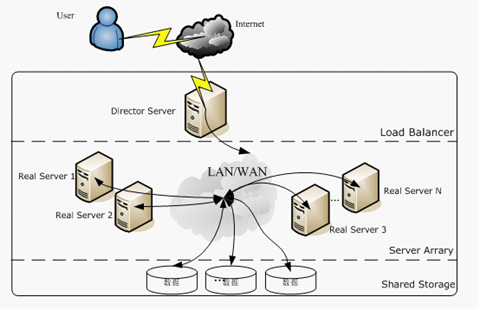腾讯Item-based CF实时推荐算法

算法设计

“实时”、“精确”和“大”挑战对推荐算法提出了多种要求。“准确”要求TencentRec尽可能提高推荐质量，“实时”和“大”则要求算法易于训练和扩展，以适应分布式系统。这两个要求在某种程度上有冲突。为了快速获得大量数据的建议，建议算法应该使用简单，但这往往会降低建议的总体质量。一个高效的推荐系统必须兼顾这两个方面，为了实现可扩展的推荐，实时推荐系统中常用的方法是数据采样，它可以在有限的计算资源内产生推荐，但往往会降低推荐的准确性。我们非常希望使用所有可用的数据来提供准确的建议。考虑到这一点，我们求助于能够扩展分布式推荐系统并保持其有效性的经典算法，如基于内容的算法、协同过滤算法（CF）、基于关联规则的算法（AR）和情境CTR算法。我们在TencentRec实现上述算法，以满足生产应用程序的各种需求。特别是，我们采用了一些机制使这些算法更适用于生产使用，包括应用人口统计学方法来解决数据稀疏问题，以及一些实时过滤技术来捕捉用户的实时兴趣。此外，我们还开发了一种实用的、可扩展的、基于项目的CF方法，可以很容易地在TencentRec等分布式流计算系统中实现。我们将在这里介绍我们的基于项目的实际CF算法，并以它为例说明用于生产使用的机制。

一种实用的基于项目的协同过滤方法

$$sim(i_p,i_q) = \frac{\vec{i_p}\cdot \vec{i_q}}{\left \| \vec{i_p} \right \|\left \| \vec{i_q} \right \|}=\frac{\sum_{u\in U}r_{u,p}r_{u,q}}{\sqrt{\sum r^2_{u,p}}\sqrt{\sum r^2_{u,q}}}$$

$$\hat{r}_{u,p} = \frac{\sum_{i_q \in N^k(i_p)}sim(i_p,i_q)r_{u,i_q}}{\sum_{i_q \in N^k(i_p)}sim(i_p,i_q)}$$

$$\text{co-rating}(i_p,i_q)=\min(r_{u,p},r_{u,q})$$

$$\text{sim}(i_p,i_q) = \frac{\sum_{u \in U}\min(r_{u,p},r_{u,q})}{\sqrt{\sum r_{u,p}}\sqrt{\sum r_{u,q}}}$$

$$\text{sim}(i_p,i_q) = \frac{\text{pairCount}(i_p,i_q)}{\sqrt{\text{itemCount}(i_p)}\sqrt{\text{itemCount}(i_q)}}$$

$$\text{itemCount}(i_p)=\sum r_{u,p}$$

$$\text{pairCount}(i_p,i_q)=\sum_{u \in U}\text{co-rating}(i_p,i_q)$$

$${\text{sim}(i_p,i_q)}’ = \frac{{\text{pairCount}(i_p,i_q)}’}{\sqrt{{\text{itemCount}(i_p)}’}\sqrt{{\text{itemCount}(i_q)}’}} = \frac{pairCount(i_p,i_q)+\Delta \text{co-rating}(i_p,i_q)}{\sqrt{\text{itemCount}(i_p)+\Delta r_{u_p}} \sqrt{\text{itemCount}(i_q)+\Delta r_{u_q}}}$$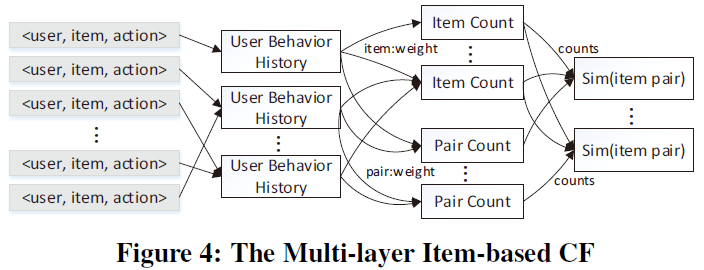$$\epsilon = \sqrt{\frac{R^2\ln(1/\delta )}{2n}}$$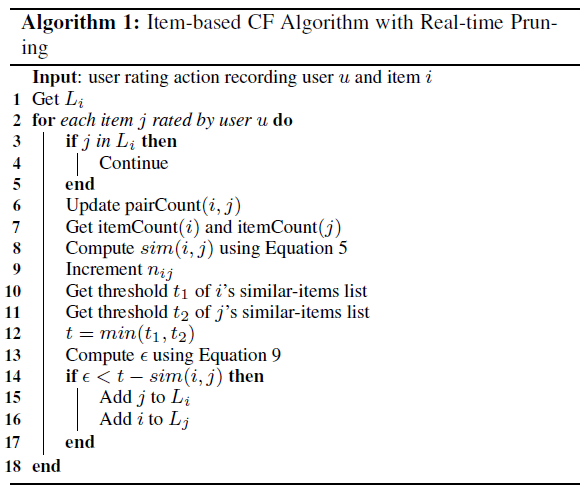解决数据稀疏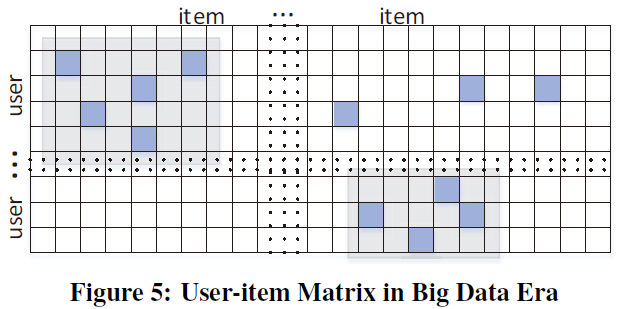实时过滤机制

$$\text{sim}(i_p,i_q) = \frac{\sum_{w \in W}\text{pairCount}_w(i_p,i_q)}{\sqrt{\sum_{w \in W}\text{itemCount}_w(i_p)}\sqrt{\sum_{w \in W}\text{itemCount}_w(i_q)}}$$

实施细节

拓扑框架

Storm中的主要概念是流，它表示一个无边界的数据元组序列。“Spout”和“Bolt”是Storm进行流处理的基本操作。“Spout”负责生成Storm集群的输入流，并将数据传递给“Bolt”。一个“Bolt”可以消耗任意数量的输入流，并以某种方式转换这些流。“Bolt”还可以发出新的流并将其传递给其他一些“Bolt”。复杂的流处理，如基于项目的CF推荐算法，可能需要多个“Spout”或“Bolt”的图，其中边缘指示流应该如何传递。该图称为“拓扑”，这是我们提交给Storm进行实时计算的内容。除非消息被杀死，否则拓扑将永远处理消息。

TencentRec作为一个通用的推荐系统，旨在为具有不同特点的不同生产应用提供推荐。这些应用程序对系统提出了不同的要求，从算法到过滤技术。例如，在新闻推荐中，新的项目不断出现，项目的生命周期很短，在这种情况下，基于项目的CF算法不太合适，而是适用于CB方法。在广告推荐中，用户是否单击广告取决于从广告图片到投放位置的任何因素，其中点击率预测算法比一般推荐算法更有效。此外，应用程序通常有自己的过滤技术，例如，推荐的项目应该是一个特定的类别或在一定范围内的价格。为了服务于所有这些应用程序，我们需要一个包含所有这些必需算法的拓扑框架，并且可以很容易地扩展以满足不同应用程序的过滤需求，如图所示：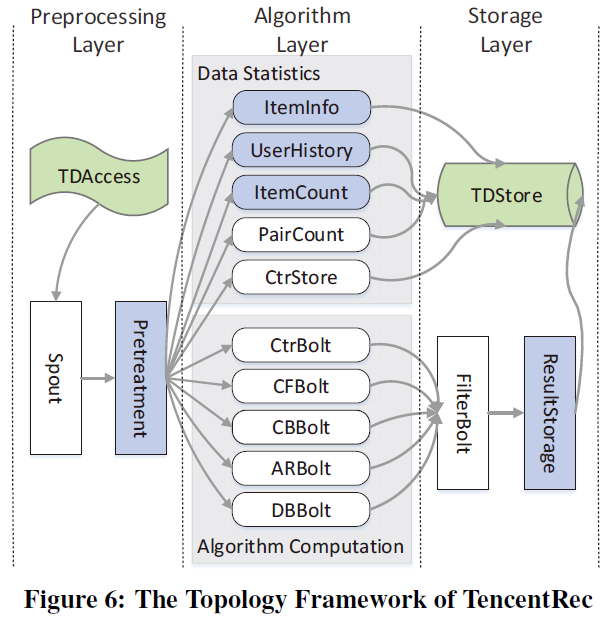TencentRec的处理可分为三大层：

• 第一层：预处理层。从TDAccess获取数据，解析原始消息，过滤不合格的数据元组，并将数据元组转换到下一层。
• 第二层：算法层。是TencentRec的主要部分，负责算法的主要计算。我们已经实现了上文中提到的一些著名的推荐算法，包括基于项目的CF、AR、CB、DB和情景CTR算法。
• 第三层：存储层。根据不同应用程序的不同规则对算法层生成的结果进行过滤，并在TDstore中更新计算结果，在响应应用程序的建议查询时，可以通过API轻松访问。

• 应用公共单元是指不同应用的公共处理步骤，如预处理和结果存储。它们在图中表示为蓝灰色矩形。
• 应用特定单元是矩形白色单位，表示特定应用（“Spout”和“Bolt”）所特有的单位。
• 算法公共单元是多个算法所需的单元，通常是数据统计，例如用蓝灰色圆角矩形表示的项计数。
• 算法特定单元是图中的白色圆角矩形单位，指特定于算法的单位，通常是特定的计算，如CFBolt和ARBolt。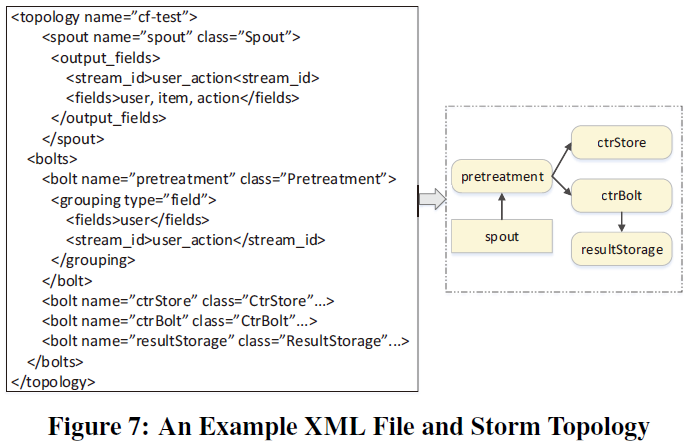部署和性能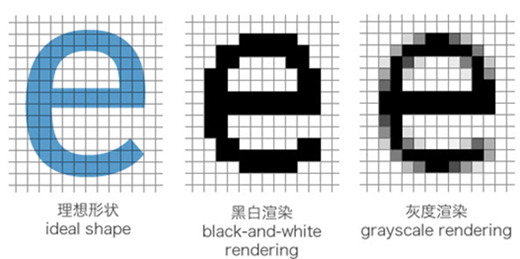网站与APP开发中的字体设置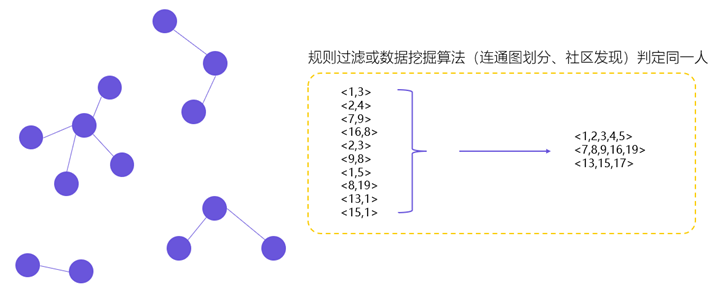用户体系搭建之ID-Mapping